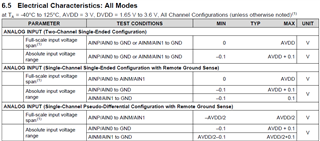If you have a related question, please click the "Ask a related question" button in the top right corner. The newly created question will be automatically linked to this question.

# ADS7142-Q1: How can I select between Full-scale input voltage and absolute input voltage?

Hello, on the data sheet

I found that there are 'full-scale input voltage' and 'absolute voltage range' for each configuration.

Is it like sub-configuration for each configuration?

How can I set with those inputs?• Hi JH,

The full-scale input range defines the differential voltage range applied between (AINP - AINN) that will create the zero-scale, and full-scale outputs.  In this case (AINP-AINN) = 0V will be zero-scale (0x000) and (AINP-AINN) = AVDD, will be full-scale (0xFFF).

The absolute input voltage range defines the absolute differential voltages that can be applied to the device, relative to GND.  The absolute differential range shows that voltages applied to the AINN/AINP pins can extend 100mV below GND, and above AVDD.  While these voltages can individually go beyond the supplies by +/-100mV, the full-scale range remains (0V to AVDD) and it's possible to saturate the output if both inputs are extended beyond the range.

Here's a few examples, assuming the single-channel, single-ended w/ remote GND mode.  AVDD = +3V:

1.) AINN = -0.1V, AINP = 2.9V, (AINP-AINN) = 3V